## Off-axis holography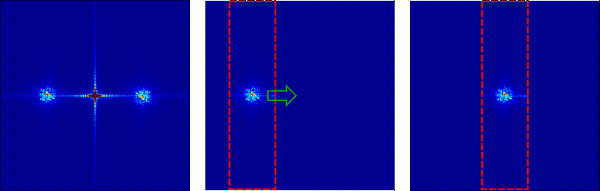Off-axis holography is a popular technique to reconstruct a hologram. It allows retrieving the amplitude and the phase of a field pattern by measuring only one image with a digital camera. It relies on an intereferometric setup with a non-zero angle between the reference beam and the signal beam and requires to numerically filter the spatial frequencies. I provida Matlab and Python example codes.

This technique was initially presented in . The schematic of the experimental setup is shown in Fig. 1. The idea is to make interfere the unknown "signal" wavefront of complex field $E_s$ with a plane wave of amplitude $$E_0$$ with a given angle $$\theta$$ between the direction of propagation of the two beams. We assume that $$E_s$$ and $$E_s$$ are in the same state of polarization.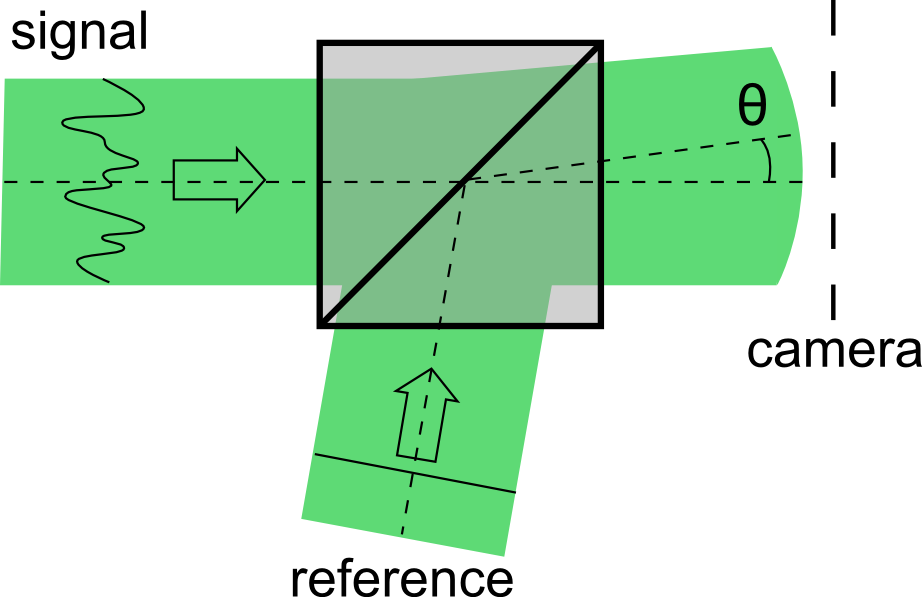Figure 1. Schematic of the setup.

The intensity measured by the camera reads:

$$I=\underbrace{\left|E_0\right|^2+\left|E_s(x,y))\right|^2}_{\textrm{order 0}}+\underbrace{E_0 E_s^*(x,y)e^{ik \sin\theta x}}_{\textrm{order +1}}+ \underbrace{E_0^* E_s(x,y)e^{-ik \sin\theta x}}_{\textrm{order -1}}$$

The three terms correspond to signals centered around the spatial frequencies 0, $$\nu = \frac{k \sin(\theta))}{2\pi}$$ and $$-\nu$$. If the spatial frequency $$\nu$$ is larger than the maximal spatial frequency in the signal to reconstruct, the Fourier transform of the measured intensity pattern shows three components corresponding orders 0, +1 and -1 that do not overlap. We note that since $E_0$ is constant, the order -1 is directly proportional to the complex field $$E_s$$ we want to retrieve but simply shifted by the spatial frequency $$\nu$$. The idea is then to numerically select the order -1 in the Fourier domain and center the signal around the zero frequency. The inverse Fourier transform then directly gives access to the complex image $$E_s$$. The steps of this process are illustrated in Fig. 2 and Fig. 3.

The following images were obtained with the Matlab code attached to this article and used to simulate an off-axis holography measurement (the file is available at the bottom of this page, the code includes comments). For the sake of simplicity, in this example, we try to recover an image with a flat amplitude and with a random phase pattern, similar to the phase of speckle pattern [Fig 2.a.]. After interference with the tilted plane wave [Fig 2.b.], we calculate the intensity pattern as it would be measured on the camera [Fig 2.c.].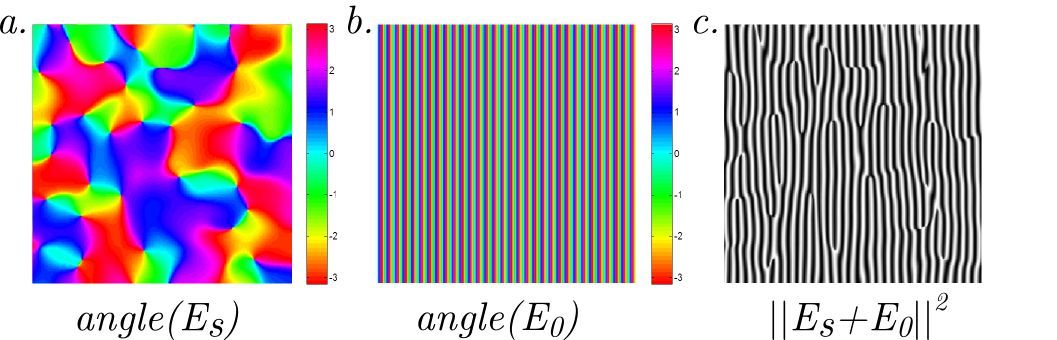Figure 2. Phase wavefront to measure (a), tilted plane wave reference (b), and resulting interference pattern.

We then calculate the Fourier transform of this intensity pattern [Fig 3.a.]. We note that the angle of the tilted plane wave has to be high enough so that order 0 and -1 do not overlap. We then select only the -1 order [Fig 3.b.] and shift it around the zero frequency [Fig 3.b.].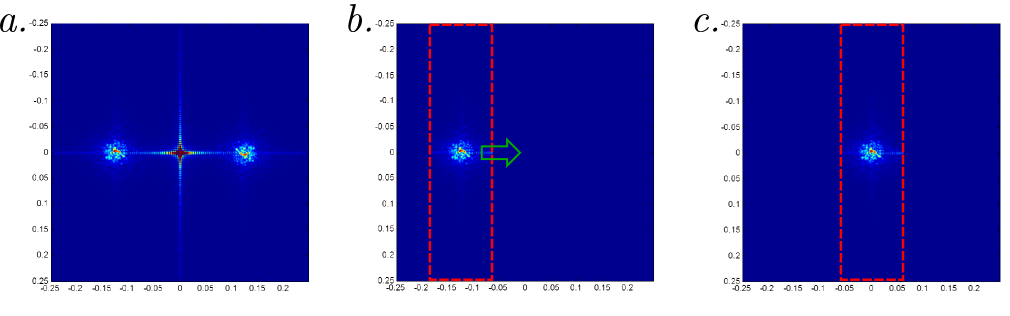Figure 3. Numerical spatial frequency filtering. (a) Complete Fourier transform of the intensity pattern. (b) Fourier transform filtered to keep only the -1 order. (c) Signal shifted around the zero spatial frequency.

The reconstructed complex field is obtained by calculating the inverse Fourier transform of the last step. We compare in Fig 4. the phase of the in initial image and the phase of the recovered one.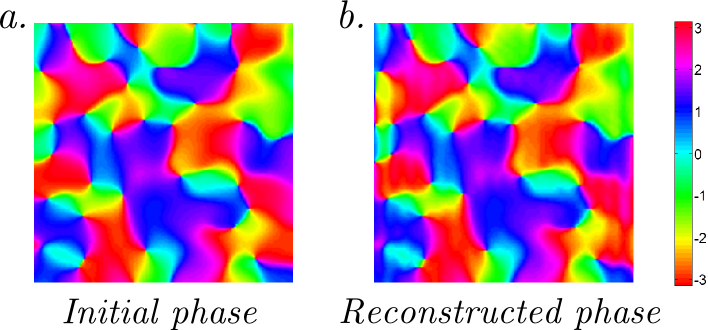Figure 4. Comparison between the actual phase pattern (a) and the reconstructed one (b).

This technique allows performing a one-shot measurement of the complex field for one state of polarization at the cost of a loss of resolution due to the need to resolve the fringes inside one speckle grain. Such a technique can be extended to obtain a full image for both polarizations by using two reference beams with orthogonal polarizations with tilt angles $$\theta_x$$ and $$\theta_y$$ in two different directions.

You can find the code on my GitHub account:

 E. Cuche et al., Opt. Express, 39, (2000)

Created by sebastien.popoff on 10/10/2013